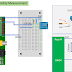## Top Ad unit 728 × 90

Temperature & Humidity Measurement

Firstly let look on circuit wiring.

DHT11 Communication:

1-> Send signal to trigger measurement action for DHT11.
Step 1 : Send start signal
- Rasp Pi set DATA as ouput, then pull DATA to low state (0) during 18ms à DHT11 understand that, RaspPi want to measure temperature and humidity.
- Rasp Pi get DATA to high state(1) then set DATA pin as input.
- After 20-40uSec DHT will pull RaspPi Data pin to low state. If >40uSec Data pin stall in the high state mean that still cannot contacted with DHT yet.
- Data pin will keep low state during 80us then DHT will pull to high state after 80uSec. By monitor Data pin, RaspPi can know that communication is ok or not. If signal raising up after 80uSec mean that DHT complete send data to Raspberry.
Step 2: Read data in DHT11.
- DHT11 will return temperature and humidity data as 5 bytes:
+ Byte 1 integer value of humidity.
+ Byte 2 decimal value of the humidity.
+ Byte 3 integer value of the temperature.
+ Byte 4 decimal value of the temperature.
+ Byte 5 check the total value.
- If Byte 5=Byte1+Byte2+Byte3+Byte4, then measurement process is correct, otherwise it was wrong.
- Read data: after communicated with DHT11 , DHT11 will send 40bit 0 or 1 continuous to RaspPi (=5 bytes)How to recognize Data signal:
After signal come to low state we waiting for Data of RaspPi pull to high state by DHT. If DATA pin in high state during 26~28uSec mean that data bit is “0”  elseif high state during 70uSec is “1”. Therefore when we coding we detected DATA raising pulse then waiting for 50uSec if value = 0 à Bit = “0” otherwise Bit =“1”
You also need to install pigpio library: http://xfunpi.blogspot.ca/2016/12/how-to-install-raspberry-pi-as-os.html

Video: Ongoing...[Will update on youtube channel: funPi]
Source Code:

import time
import atexit
import pigpio
class sensor:
def __init__(self, pi, gpio, LED=None, power=None):

self.pi = pi
self.gpio = gpio
self.LED = LED
self.power = power
if power is not None:
pi.write(power, 1) # Switch sensor on.
time.sleep(2)
self.powered = True
self.cb = None
atexit.register(self.cancel)
self.bad_SM = 0 # Short message count.
self.bad_MM = 0 # Missing message count.
self.bad_SR = 0 # Sensor reset count.
# Power cycle if timeout > MAX_TIMEOUTS.
self.no_response = 0
self.MAX_NO_RESPONSE = 2
self.rhum = -999
self.temp = -999
self.tov = None
self.high_tick = 0
self.bit = 40
pi.set_pull_up_down(gpio, pigpio.PUD_OFF)
pi.set_watchdog(gpio, 0) # Kill any watchdogs.
self.cb = pi.callback(gpio, pigpio.EITHER_EDGE, self._cb)
def _cb(self, gpio, level, tick):
"""
Accumulate the 40 data bits.  Format into 5 bytes, humidity high,
humidity low, temperature high, temperature low, checksum.
"""
diff = pigpio.tickDiff(self.high_tick, tick)
if level == 0:
# Edge length determines if bit is 1 or 0.
if diff >= 50:
val = 1
if diff >= 200: # Bad bit?
self.CS = 256 # Force bad checksum.
else:
val = 0
if self.bit >= 40: # Message complete.
self.bit = 40
elif self.bit >= 32: # In checksum byte.
self.CS  = (self.CS<<1)  + val
if self.bit == 39:
self.pi.set_watchdog(self.gpio, 0)
self.no_response = 0
total = self.hH + self.hL + self.tH + self.tL
if (total & 255) == self.CS: # Is checksum ok?
self.rhum = ((self.hH<<8) + self.hL) * 0.1
if self.tH & 128: # Negative temperature.
mult = -0.1
self.tH = self.tH & 127
else:
mult = 0.1
self.temp = ((self.tH<<8) + self.tL) * mult
self.tov = time.time()
if self.LED is not None:
self.pi.write(self.LED, 0)
else:
elif self.bit >=24: # in temp low byte
self.tL = (self.tL<<1) + val
elif self.bit >=16: # in temp high byte
self.tH = (self.tH<<1) + val
elif self.bit >= 8: # in humidity low byte
self.hL = (self.hL<<1) + val
elif self.bit >= 0: # in humidity high byte
self.hH = (self.hH<<1) + val
pass
self.bit += 1
elif level == 1:
self.high_tick = tick
if diff > 250000:
self.bit = -2
self.hH = 0
self.hL = 0
self.tH = 0
self.tL = 0
self.CS = 0
else: # level == pigpio.TIMEOUT:
self.pi.set_watchdog(self.gpio, 0)
if self.bit < 8:       # Too few data bits received.
self.bad_MM += 1    # Bump missing message count.
self.no_response += 1
if self.no_response > self.MAX_NO_RESPONSE:
self.no_response = 0
self.bad_SR += 1 # Bump sensor reset count.
if self.power is not None:
self.powered = False
self.pi.write(self.power, 0)
time.sleep(2)
self.pi.write(self.power, 1)
time.sleep(2)
self.powered = True
elif self.bit < 39:    # Short message receieved.
self.bad_SM += 1    # Bump short message count.
self.no_response = 0
self.no_response = 0
def temperature(self):
"""Return current temperature."""
return self.temp
def humidity(self):
"""Return current relative humidity."""
return self.rhum
def staleness(self):
if self.tov is not None:
return time.time() - self.tov
else:
return -999
def short_message(self):
"""Return count of short messages."""
def missing_message(self):
"""Return count of missing messages."""
def sensor_resets(self):
"""Return count of power cycles because of sensor hangs."""
def trigger(self):
"""Trigger a new relative humidity and temperature reading."""
if self.powered:
if self.LED is not None:
self.pi.write(self.LED, 1)
self.pi.write(self.gpio, pigpio.LOW)
time.sleep(0.017) # 17 ms
self.pi.set_mode(self.gpio, pigpio.INPUT)
self.pi.set_watchdog(self.gpio, 200)
def cancel(self):
"""Cancel the DHT22 sensor."""
self.pi.set_watchdog(self.gpio, 0)
if self.cb != None:
self.cb.cancel()
self.cb = None
if __name__ == "__main__":
import time
import pigpio
import DHT22
# Intervals of about 2 seconds or less will eventually hang the DHT22.
INTERVAL=3
pi = pigpio.pi()
s = DHT22.sensor(pi, 22, LED=16, power=8)
r = 0
while True:
r += 1
s.trigger()
time.sleep(0.2)
print("{} {} {} {:3.2f} {} {} {} {}".format(
r, s.humidity(), s.temperature(), s.staleness(),
s.sensor_resets()))
time.sleep(next_reading-time.time()) # Overall INTERVAL second polling.
s.cancel()
pi.stop()

Temperature and Humidity Measurement by Raspberry on DHT11Reviewed by Unknown on December 15, 2016 Rating: 5